# 12.4 Rotation of axes  (Page 5/8)

 Page 5 / 8

Identify the conic for each of the following without rotating axes.

1. ${x}^{2}-9xy+3{y}^{2}-12=0$
2. $10{x}^{2}-9xy+4{y}^{2}-4=0$
1. hyperbola
2. ellipse

Access this online resource for additional instruction and practice with conic sections and rotation of axes.

## Key equations

 General Form equation of a conic section $A{x}^{2}+Bxy+C{y}^{2}+Dx+Ey+F=0$ Rotation of a conic section Angle of rotation

## Key concepts

• Four basic shapes can result from the intersection of a plane with a pair of right circular cones connected tail to tail. They include an ellipse, a circle, a hyperbola, and a parabola.
• A nondegenerate conic section has the general form $\text{\hspace{0.17em}}A{x}^{2}+Bxy+C{y}^{2}+Dx+Ey+F=0\text{\hspace{0.17em}}$ where $\text{\hspace{0.17em}}A,B\text{\hspace{0.17em}}$ and $\text{\hspace{0.17em}}C\text{\hspace{0.17em}}$ are not all zero. The values of $\text{\hspace{0.17em}}A,B,$ and $\text{\hspace{0.17em}}C\text{\hspace{0.17em}}$ determine the type of conic. See [link] .
• Equations of conic sections with an $\text{\hspace{0.17em}}xy\text{\hspace{0.17em}}$ term have been rotated about the origin. See [link] .
• The general form can be transformed into an equation in the $\text{\hspace{0.17em}}{x}^{\prime }\text{\hspace{0.17em}}$ and $\text{\hspace{0.17em}}{y}^{\prime }\text{\hspace{0.17em}}$ coordinate system without the $\text{\hspace{0.17em}}{x}^{\prime }{y}^{\prime }\text{\hspace{0.17em}}$ term. See [link] and [link] .
• An expression is described as invariant if it remains unchanged after rotating. Because the discriminant is invariant, observing it enables us to identify the conic section. See [link] .

## Verbal

What effect does the $\text{\hspace{0.17em}}xy\text{\hspace{0.17em}}$ term have on the graph of a conic section?

The $\text{\hspace{0.17em}}xy\text{\hspace{0.17em}}$ term causes a rotation of the graph to occur.

If the equation of a conic section is written in the form $\text{\hspace{0.17em}}A{x}^{2}+B{y}^{2}+Cx+Dy+E=0\text{\hspace{0.17em}}$ and $\text{\hspace{0.17em}}AB=0,$ what can we conclude?

If the equation of a conic section is written in the form $\text{\hspace{0.17em}}A{x}^{2}+Bxy+C{y}^{2}+Dx+Ey+F=0,$ and $\text{\hspace{0.17em}}{B}^{2}-4AC>0,$ what can we conclude?

The conic section is a hyperbola.

Given the equation $\text{\hspace{0.17em}}a{x}^{2}+4x+3{y}^{2}-12=0,$ what can we conclude if $\text{\hspace{0.17em}}a>0?$

For the equation $\text{\hspace{0.17em}}A{x}^{2}+Bxy+C{y}^{2}+Dx+Ey+F=0,$ the value of $\text{\hspace{0.17em}}\theta \text{\hspace{0.17em}}$ that satisfies $\text{\hspace{0.17em}}\mathrm{cot}\left(2\theta \right)=\frac{A-C}{B}\text{\hspace{0.17em}}$ gives us what information?

It gives the angle of rotation of the axes in order to eliminate the $\text{\hspace{0.17em}}xy\text{\hspace{0.17em}}$ term.

## Algebraic

For the following exercises, determine which conic section is represented based on the given equation.

$9{x}^{2}+4{y}^{2}+72x+36y-500=0$

${x}^{2}-10x+4y-10=0$

$AB=0,$ parabola

$2{x}^{2}-2{y}^{2}+4x-6y-2=0$

$4{x}^{2}-{y}^{2}+8x-1=0$

$AB=-4<0,$ hyperbola

$4{y}^{2}-5x+9y+1=0$

$2{x}^{2}+3{y}^{2}-8x-12y+2=0$

$AB=6>0,$ ellipse

$4{x}^{2}+9xy+4{y}^{2}-36y-125=0$

$3{x}^{2}+6xy+3{y}^{2}-36y-125=0$

${B}^{2}-4AC=0,$ parabola

$-3{x}^{2}+3\sqrt{3}xy-4{y}^{2}+9=0$

$2{x}^{2}+4\sqrt{3}xy+6{y}^{2}-6x-3=0$

${B}^{2}-4AC=0,$ parabola

$-{x}^{2}+4\sqrt{2}xy+2{y}^{2}-2y+1=0$

$8{x}^{2}+4\sqrt{2}xy+4{y}^{2}-10x+1=0$

${B}^{2}-4AC=-96<0,$ ellipse

For the following exercises, find a new representation of the given equation after rotating through the given angle.

$3{x}^{2}+xy+3{y}^{2}-5=0,\theta =45°$

$4{x}^{2}-xy+4{y}^{2}-2=0,\theta =45°$

$7{{x}^{\prime }}^{2}+9{{y}^{\prime }}^{2}-4=0$

$2{x}^{2}+8xy-1=0,\theta =30°$

$-2{x}^{2}+8xy+1=0,\theta =45°$

$3{{x}^{\prime }}^{2}+2{x}^{\prime }{y}^{\prime }-5{{y}^{\prime }}^{2}+1=0$

$4{x}^{2}+\sqrt{2}xy+4{y}^{2}+y+2=0,\theta =45°$

For the following exercises, determine the angle $\text{\hspace{0.17em}}\theta \text{\hspace{0.17em}}$ that will eliminate the $\text{\hspace{0.17em}}xy\text{\hspace{0.17em}}$ term and write the corresponding equation without the $\text{\hspace{0.17em}}xy\text{\hspace{0.17em}}$ term.

${x}^{2}+3\sqrt{3}xy+4{y}^{2}+y-2=0$

$\theta ={60}^{\circ },11{{x}^{\prime }}^{2}-{{y}^{\prime }}^{2}+\sqrt{3}{x}^{\prime }+{y}^{\prime }-4=0$

$4{x}^{2}+2\sqrt{3}xy+6{y}^{2}+y-2=0$

$9{x}^{2}-3\sqrt{3}xy+6{y}^{2}+4y-3=0$

$\theta ={150}^{\circ },21{{x}^{\prime }}^{2}+9{{y}^{\prime }}^{2}+4{x}^{\prime }-4\sqrt{3}{y}^{\prime }-6=0$

$-3{x}^{2}-\sqrt{3}xy-2{y}^{2}-x=0$

$16{x}^{2}+24xy+9{y}^{2}+6x-6y+2=0$

$\theta \approx {36.9}^{\circ },125{{x}^{\prime }}^{2}+6{x}^{\prime }-42{y}^{\prime }+10=0$

${x}^{2}+4xy+4{y}^{2}+3x-2=0$

${x}^{2}+4xy+{y}^{2}-2x+1=0$

$\theta ={45}^{\circ },3{{x}^{\prime }}^{2}-{{y}^{\prime }}^{2}-\sqrt{2}{x}^{\prime }+\sqrt{2}{y}^{\prime }+1=0$

$4{x}^{2}-2\sqrt{3}xy+6{y}^{2}-1=0$

## Graphical

For the following exercises, rotate through the given angle based on the given equation. Give the new equation and graph the original and rotated equation.

$y=-{x}^{2},\theta =-{45}^{\circ }$

$\frac{\sqrt{2}}{2}\left({x}^{\prime }+{y}^{\prime }\right)=\frac{1}{2}{\left({x}^{\prime }-{y}^{\prime }\right)}^{2}$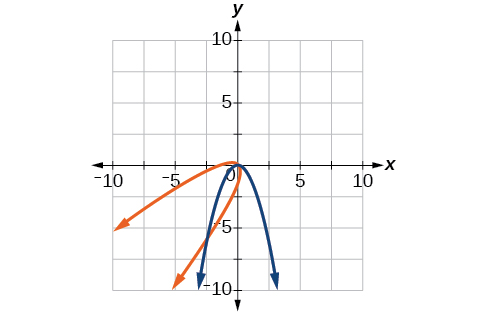$x={y}^{2},\theta ={45}^{\circ }$

$\frac{{x}^{2}}{4}+\frac{{y}^{2}}{1}=1,\theta ={45}^{\circ }$

$\frac{{\left({x}^{\prime }-{y}^{\prime }\right)}^{2}}{8}+\frac{{\left({x}^{\prime }+{y}^{\prime }\right)}^{2}}{2}=1$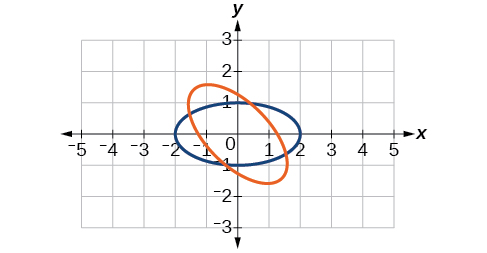$\frac{{y}^{2}}{16}+\frac{{x}^{2}}{9}=1,\theta ={45}^{\circ }$

${y}^{2}-{x}^{2}=1,\theta ={45}^{\circ }$

$\frac{{\left({x}^{\prime }+{y}^{\prime }\right)}^{2}}{2}-\frac{{\left({x}^{\prime }-{y}^{\prime }\right)}^{2}}{2}=1$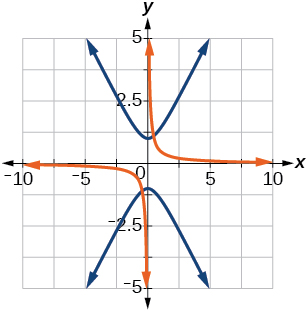$y=\frac{{x}^{2}}{2},\theta ={30}^{\circ }$

$x={\left(y-1\right)}^{2},\theta ={30}^{\circ }$

$\frac{\sqrt{3}}{2}{x}^{\prime }-\frac{1}{2}{y}^{\prime }={\left(\frac{1}{2}{x}^{\prime }+\frac{\sqrt{3}}{2}{y}^{\prime }-1\right)}^{2}$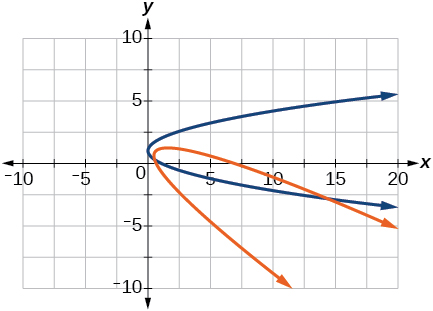$\frac{{x}^{2}}{9}+\frac{{y}^{2}}{4}=1,\theta ={30}^{\circ }$

For the following exercises, graph the equation relative to the $\text{\hspace{0.17em}}{x}^{\prime }{y}^{\prime }\text{\hspace{0.17em}}$ system in which the equation has no $\text{\hspace{0.17em}}{x}^{\prime }{y}^{\prime }\text{\hspace{0.17em}}$ term.

$xy=9$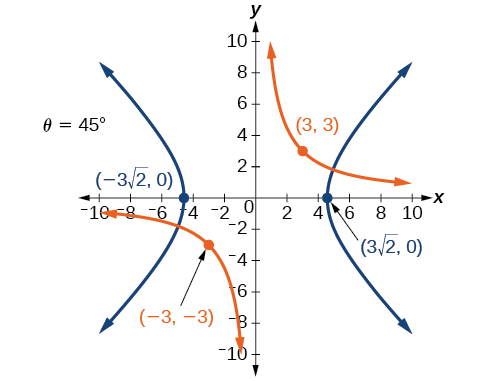${x}^{2}+10xy+{y}^{2}-6=0$

${x}^{2}-10xy+{y}^{2}-24=0$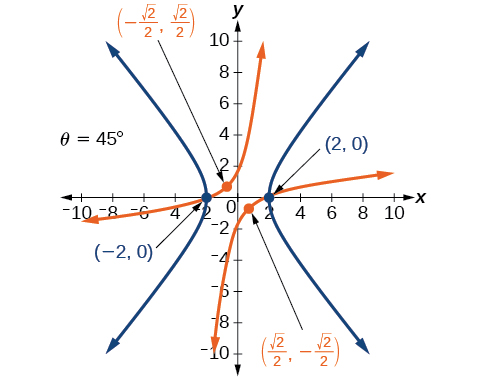$4{x}^{2}-3\sqrt{3}xy+{y}^{2}-22=0$

$6{x}^{2}+2\sqrt{3}xy+4{y}^{2}-21=0$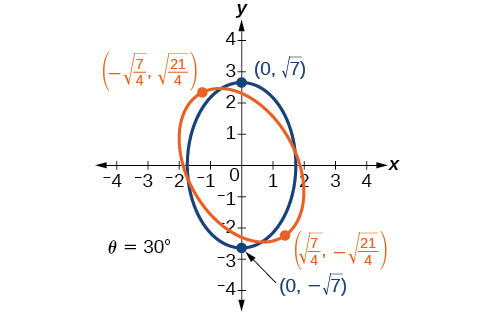$11{x}^{2}+10\sqrt{3}xy+{y}^{2}-64=0$

$21{x}^{2}+2\sqrt{3}xy+19{y}^{2}-18=0$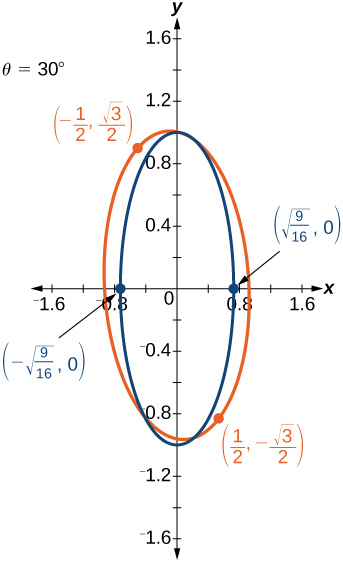$16{x}^{2}+24xy+9{y}^{2}-130x+90y=0$

$16{x}^{2}+24xy+9{y}^{2}-60x+80y=0$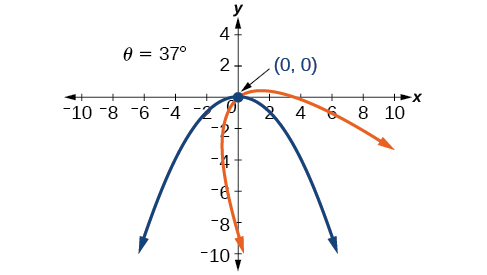$13{x}^{2}-6\sqrt{3}xy+7{y}^{2}-16=0$

$4{x}^{2}-4xy+{y}^{2}-8\sqrt{5}x-16\sqrt{5}y=0$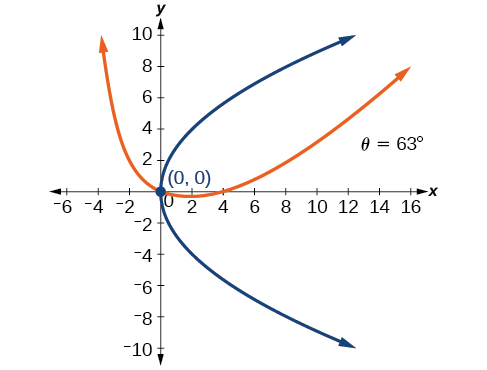For the following exercises, determine the angle of rotation in order to eliminate the $\text{\hspace{0.17em}}xy\text{\hspace{0.17em}}$ term. Then graph the new set of axes.

$6{x}^{2}-5\sqrt{3}xy+{y}^{2}+10x-12y=0$

$6{x}^{2}-5xy+6{y}^{2}+20x-y=0$

$\theta ={45}^{\circ }$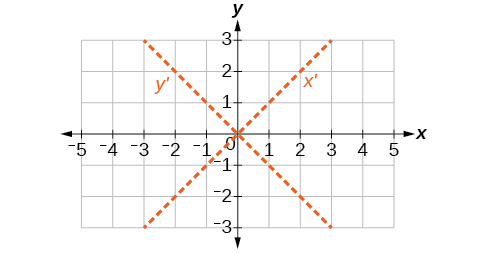$6{x}^{2}-8\sqrt{3}xy+14{y}^{2}+10x-3y=0$

$4{x}^{2}+6\sqrt{3}xy+10{y}^{2}+20x-40y=0$

$\theta ={60}^{\circ }$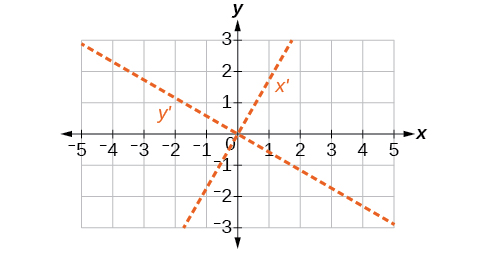$8{x}^{2}+3xy+4{y}^{2}+2x-4=0$

$16{x}^{2}+24xy+9{y}^{2}+20x-44y=0$

$\theta \approx {36.9}^{\circ }$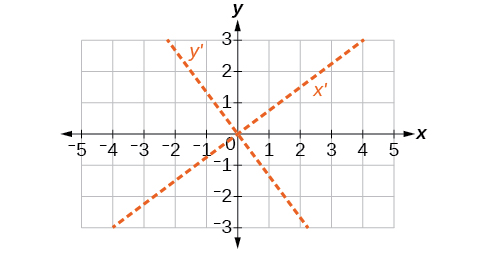For the following exercises, determine the value of $\text{\hspace{0.17em}}k\text{\hspace{0.17em}}$ based on the given equation.

Given $\text{\hspace{0.17em}}4{x}^{2}+kxy+16{y}^{2}+8x+24y-48=0,$ find $\text{\hspace{0.17em}}k\text{\hspace{0.17em}}$ for the graph to be a parabola.

Given $\text{\hspace{0.17em}}2{x}^{2}+kxy+12{y}^{2}+10x-16y+28=0,$ find $\text{\hspace{0.17em}}k\text{\hspace{0.17em}}$ for the graph to be an ellipse.

$-4\sqrt{6}

Given $\text{\hspace{0.17em}}3{x}^{2}+kxy+4{y}^{2}-6x+20y+128=0,$ find $\text{\hspace{0.17em}}k\text{\hspace{0.17em}}$ for the graph to be a hyperbola.

Given $\text{\hspace{0.17em}}k{x}^{2}+8xy+8{y}^{2}-12x+16y+18=0,$ find $\text{\hspace{0.17em}}k\text{\hspace{0.17em}}$ for the graph to be a parabola.

$k=2$

Given $\text{\hspace{0.17em}}6{x}^{2}+12xy+k{y}^{2}+16x+10y+4=0,$ find $\text{\hspace{0.17em}}k\text{\hspace{0.17em}}$ for the graph to be an ellipse.

(1+cosA+IsinA)(1+cosB+isinB)/(cos@+isin@)(cos$+isin$)
hatdog
Mark
how we can draw three triangles of distinctly different shapes. All the angles will be cutt off each triangle and placed side by side with vertices touching
bsc F. y algebra and trigonometry pepper 2
given that x= 3/5 find sin 3x
4
DB
remove any signs and collect terms of -2(8a-3b-c)
-16a+6b+2c
Will
Joeval
(x2-2x+8)-4(x2-3x+5)
sorry
Miranda
x²-2x+9-4x²+12x-20 -3x²+10x+11
Miranda
x²-2x+9-4x²+12x-20 -3x²+10x+11
Miranda
(X2-2X+8)-4(X2-3X+5)=0 ?
master
The anwser is imaginary number if you want to know The anwser of the expression you must arrange The expression and use quadratic formula To find the answer
master
The anwser is imaginary number if you want to know The anwser of the expression you must arrange The expression and use quadratic formula To find the answer
master
Y
master
master
Soo sorry (5±Root11* i)/3
master
Mukhtar
2x²-6x+1=0
Ife
explain and give four example of hyperbolic function
What is the correct rational algebraic expression of the given "a fraction whose denominator is 10 more than the numerator y?
y/y+10
Mr
Find nth derivative of eax sin (bx + c).
Find area common to the parabola y2 = 4ax and x2 = 4ay.
Anurag
y2=4ax= y=4ax/2. y=2ax
akash
A rectangular garden is 25ft wide. if its area is 1125ft, what is the length of the garden
to find the length I divide the area by the wide wich means 1125ft/25ft=45
Miranda
thanks
Jhovie
What do you call a relation where each element in the domain is related to only one value in the range by some rules?
A banana.
Yaona
given 4cot thither +3=0and 0°<thither <180° use a sketch to determine the value of the following a)cos thither
what are you up to?
nothing up todat yet
Miranda
hi
jai
hello
jai
Miranda Drice
jai
aap konsi country se ho
jai
which language is that
Miranda
I am living in india
jai
good
Miranda
what is the formula for calculating algebraic
I think the formula for calculating algebraic is the statement of the equality of two expression stimulate by a set of addition, multiplication, soustraction, division, raising to a power and extraction of Root. U believe by having those in the equation you will be in measure to calculate it
Miranda
state and prove Cayley hamilton therom
hello
Propessor
hi
Miranda
the Cayley hamilton Theorem state if A is a square matrix and if f(x) is its characterics polynomial then f(x)=0 in another ways evey square matrix is a root of its chatacteristics polynomial.
Miranda
hi
jai
hi Miranda
jai
thanks
Propessor
welcome
jai

#### Get Jobilize Job Search Mobile App in your pocket Now!ByByBy Ali SidBy OpenStaxBy Christine ZeelieBy Angela JanuaryBy Subramanian DivyaBy John GabrieliBy Lakeima RobertsBy RhodesBy Kevin MoquinBy